# Complex and semantic figure composition¶

Warning

This tutorial documents experimental / provisional API. We are releasing this in v3.3 to get user feedback. We may make breaking changes in future versions with no warning.

Laying out Axes in a Figure in a non uniform grid can be both tedious and verbose. For dense, even grids we have Figure.subplots but for more complex layouts, such as Axes that span multiple columns / rows of the layout or leave some areas of the Figure blank, you can use gridspec.GridSpec (see Customizing Figure Layouts Using GridSpec and Other Functions) or manually place your axes. Figure.subplot_mosaic aims to provide an interface to visually lay out your axes (as either ASCII art or nested lists) to streamline this process.

This interface naturally supports naming your axes. Figure.subplot_mosaic returns a dictionary keyed on the labels used to lay out the Figure. By returning data structures with names, it is easier to write plotting code that is independent of the Figure layout.

This is inspired by a proposed MEP and the patchwork library for R. While we do not implement the operator overloading style, we do provide a Pythonic API for specifying (nested) Axes layouts.

import matplotlib.pyplot as plt
import numpy as np

# Helper function used for visualization in the following examples
def identify_axes(ax_dict, fontsize=48):
"""
Helper to identify the Axes in the examples below.

Draws the label in a large font in the center of the Axes.

Parameters
----------
ax_dict : dict[str, Axes]
Mapping between the title / label and the Axes.
fontsize : int, optional
How big the label should be.
"""
kw = dict(ha="center", va="center", fontsize=fontsize, color="darkgrey")
for k, ax in ax_dict.items():
ax.text(0.5, 0.5, k, transform=ax.transAxes, **kw)


If we want a 2x2 grid we can use Figure.subplots which returns a 2D array of axes.Axes which we can index into to do our plotting.

np.random.seed(19680801)
hist_data = np.random.randn(1_500)

fig = plt.figure(constrained_layout=True)
ax_array = fig.subplots(2, 2, squeeze=False)

ax_array[0, 0].bar(["a", "b", "c"], [5, 7, 9])
ax_array[0, 1].plot([1, 2, 3])
ax_array[1, 0].hist(hist_data, bins="auto")
ax_array[1, 1].imshow([[1, 2], [2, 1]])

identify_axes(
{(j, k): a for j, r in enumerate(ax_array) for k, a in enumerate(r)},
)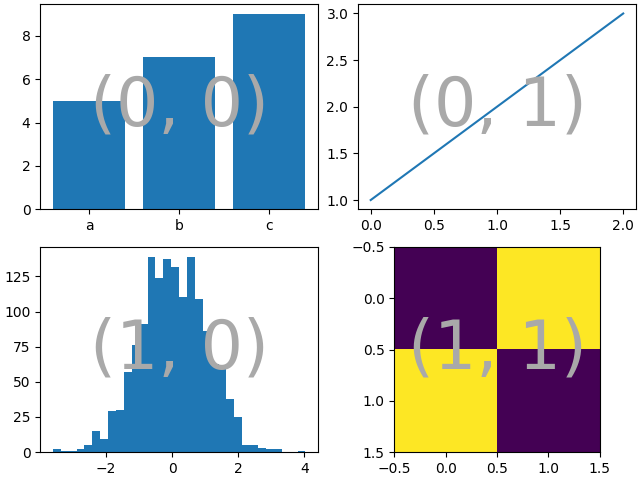Using Figure.subplot_mosaic we can produce the same mosaic but give the axes semantic names

fig = plt.figure(constrained_layout=True)
ax_dict = fig.subplot_mosaic(
[
["bar", "plot"],
["hist", "image"],
],
)
ax_dict["bar"].bar(["a", "b", "c"], [5, 7, 9])
ax_dict["plot"].plot([1, 2, 3])
ax_dict["hist"].hist(hist_data)
ax_dict["image"].imshow([[1, 2], [2, 1]])
identify_axes(ax_dict)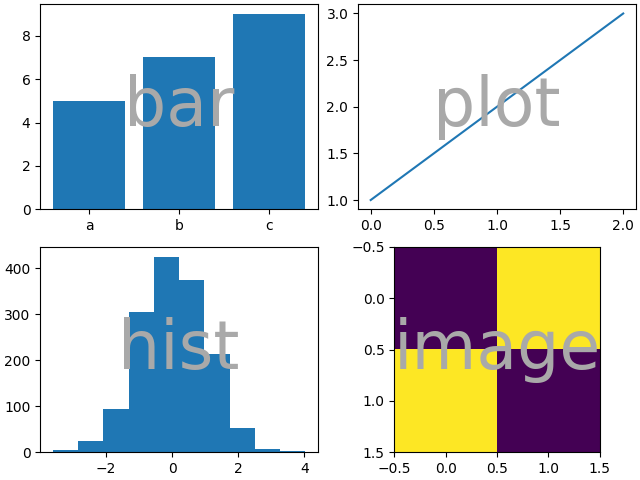A key difference between Figure.subplots and Figure.subplot_mosaic is the return value. While the former returns an array for index access, the latter returns a dictionary mapping the labels to the axes.Axes instances created

print(ax_dict)


Out:

{'bar': <AxesSubplot:label='bar'>, 'plot': <AxesSubplot:label='plot'>, 'hist': <AxesSubplot:label='hist'>, 'image': <AxesSubplot:label='image'>}


## String short-hand¶

By restricting our axes labels to single characters we can use Using we can "draw" the Axes we want as "ASCII art". The following

mosaic = """
AB
CD
"""


will give us 4 Axes laid out in a 2x2 grid and generates the same figure mosaic as above (but now labeled with {"A", "B", "C", "D"} rather than {"bar", "plot", "hist", "image"}).

fig = plt.figure(constrained_layout=True)
ax_dict = fig.subplot_mosaic(mosaic)
identify_axes(ax_dict)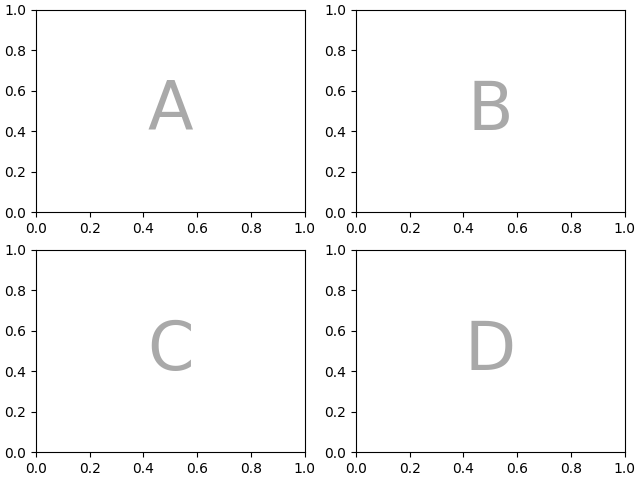Something we can do with Figure.subplot_mosaic that you can not do with Figure.subplots is specify that an Axes should span several rows or columns.

If we want to re-arrange our four Axes to have C be a horizontal span on the bottom and D be a vertical span on the right we would do

axd = plt.figure(constrained_layout=True).subplot_mosaic(
"""
ABD
CCD
"""
)
identify_axes(axd)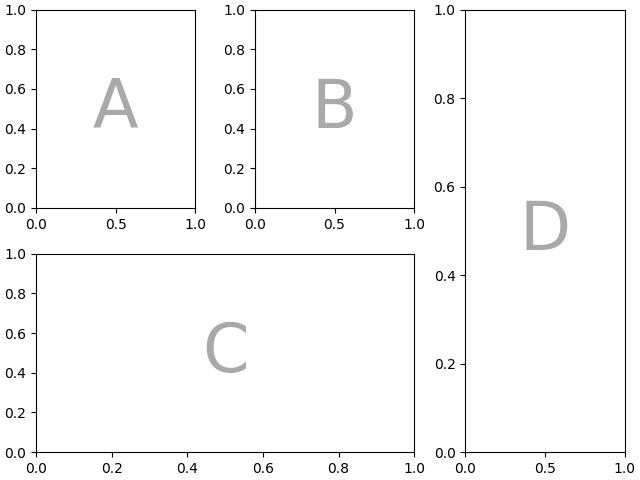If we do not want to fill in all the spaces in the Figure with Axes, we can specify some spaces in the grid to be blank

axd = plt.figure(constrained_layout=True).subplot_mosaic(
"""
A.C
BBB
.D.
"""
)
identify_axes(axd)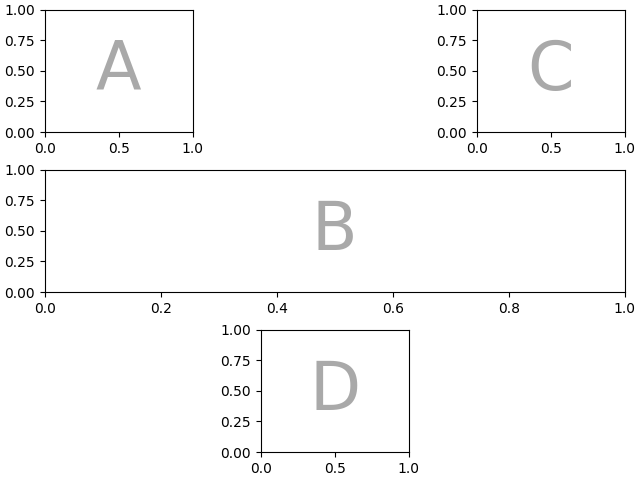If we prefer to use another character (rather than a period ".") to mark the empty space, we can use empty_sentinel to specify the character to use.

axd = plt.figure(constrained_layout=True).subplot_mosaic(
"""
aX
Xb
""",
empty_sentinel="X",
)
identify_axes(axd)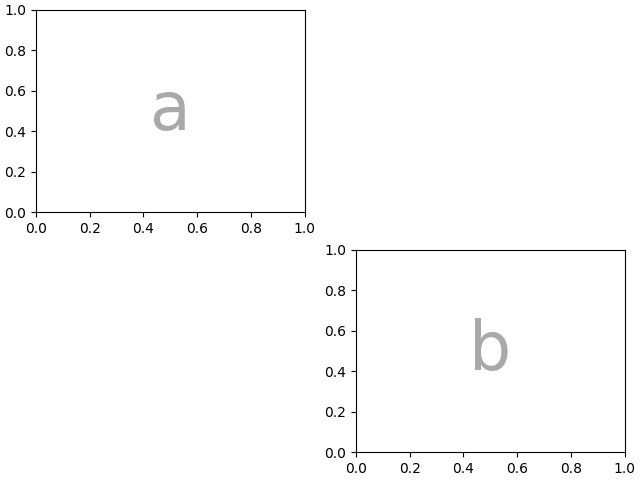Internally there is no meaning attached to the letters we use, any Unicode code point is valid!

axd = plt.figure(constrained_layout=True).subplot_mosaic(
"""αб
ℝ☢"""
)
identify_axes(axd)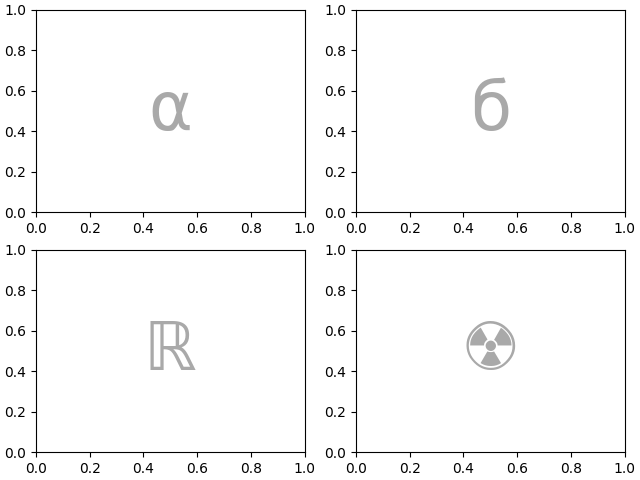It is not recommended to use white space as either a label or an empty sentinel with the string shorthand because it may be stripped while processing the input.

## Controlling mosaic and subplot creation¶

This feature is built on top of gridspec and you can pass the keyword arguments through to the underlying gridspec.GridSpec (the same as Figure.subplots).

In this case we want to use the input to specify the arrangement, but set the relative widths of the rows / columns via gridspec_kw.

axd = plt.figure(constrained_layout=True).subplot_mosaic(
"""
.a.
bAc
.d.
""",
gridspec_kw={
# set the height ratios between the rows
"height_ratios": [1, 3.5, 1],
# set the width ratios between the columns
"width_ratios": [1, 3.5, 1],
},
)
identify_axes(axd)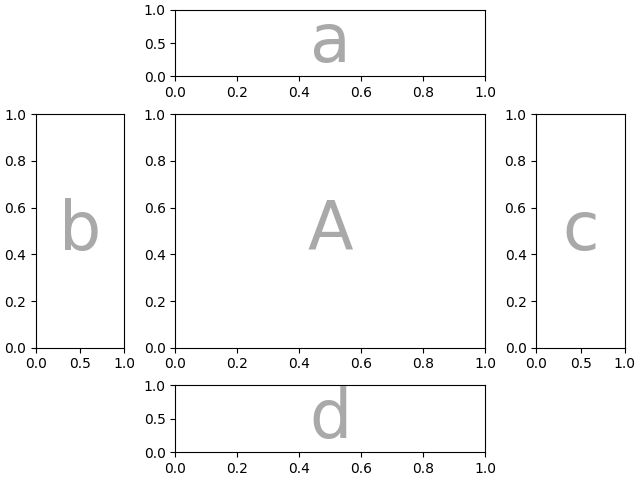Or use the {left, right, bottom, top} keyword arguments to position the overall mosaic to put multiple versions of the same mosaic in a figure

mosaic = """AA
BC"""
fig = plt.figure()
axd = fig.subplot_mosaic(
mosaic,
gridspec_kw={
"bottom": 0.25,
"top": 0.95,
"left": 0.1,
"right": 0.5,
"wspace": 0.5,
"hspace": 0.5,
},
)
identify_axes(axd)

axd = fig.subplot_mosaic(
mosaic,
gridspec_kw={
"bottom": 0.05,
"top": 0.75,
"left": 0.6,
"right": 0.95,
"wspace": 0.5,
"hspace": 0.5,
},
)
identify_axes(axd)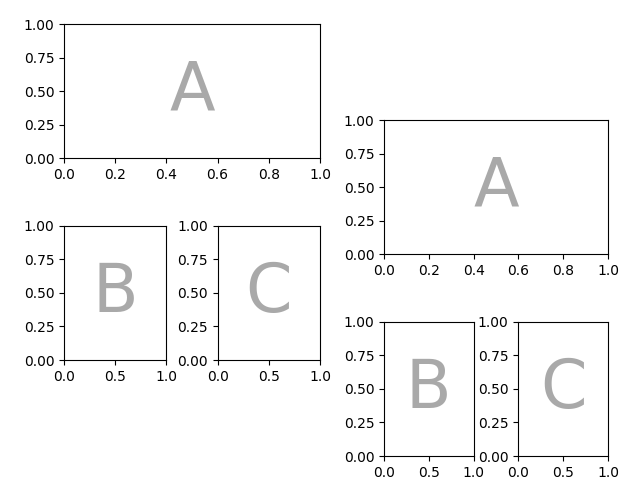Alternatively, you can use the sub-Figure functionality:

mosaic = """AA
BC"""
fig = plt.figure(constrained_layout=True)
left, right = fig.subfigures(nrows=1, ncols=2)
axd = left.subplot_mosaic(mosaic)
identify_axes(axd)

axd = right.subplot_mosaic(mosaic)
identify_axes(axd)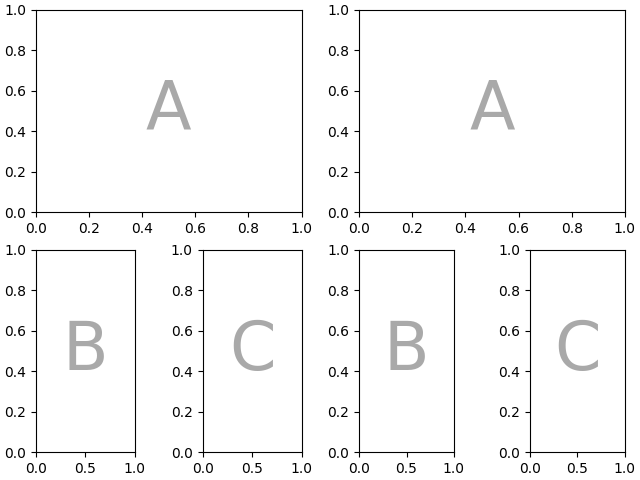We can also pass through arguments used to create the subplots (again, the same as Figure.subplots).

axd = plt.figure(constrained_layout=True).subplot_mosaic(
"AB", subplot_kw={"projection": "polar"}
)
identify_axes(axd)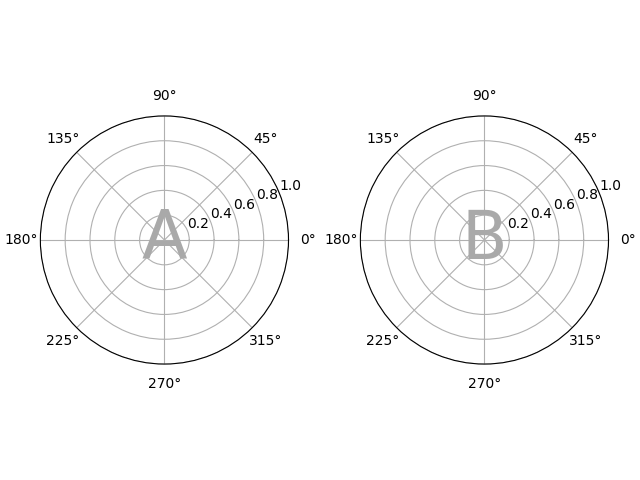## Nested List input¶

Everything we can do with the string short-hand we can also do when passing in a list (internally we convert the string shorthand to a nested list), for example using spans, blanks, and gridspec_kw:

axd = plt.figure(constrained_layout=True).subplot_mosaic(
[
["main", "zoom"],
["main", "BLANK"],
],
empty_sentinel="BLANK",
gridspec_kw={"width_ratios": [2, 1]},
)
identify_axes(axd)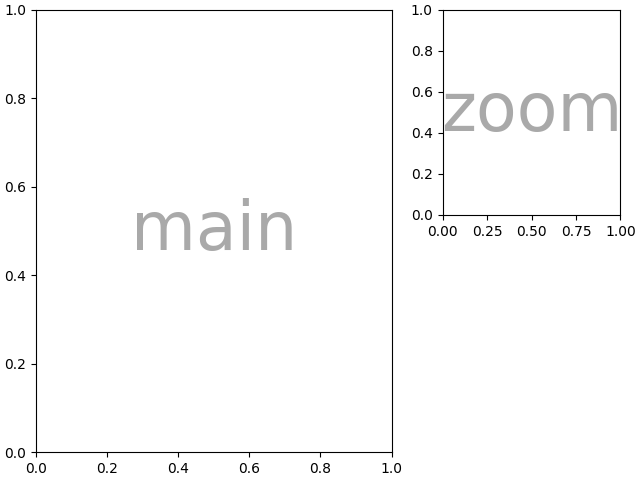In addition, using the list input we can specify nested mosaics. Any element of the inner list can be another set of nested lists:

inner = [
["inner A"],
["inner B"],
]

outer_nested_mosaic = [
["main", inner],
["bottom", "bottom"],
]
axd = plt.figure(constrained_layout=True).subplot_mosaic(
outer_nested_mosaic, empty_sentinel=None
)
identify_axes(axd, fontsize=36)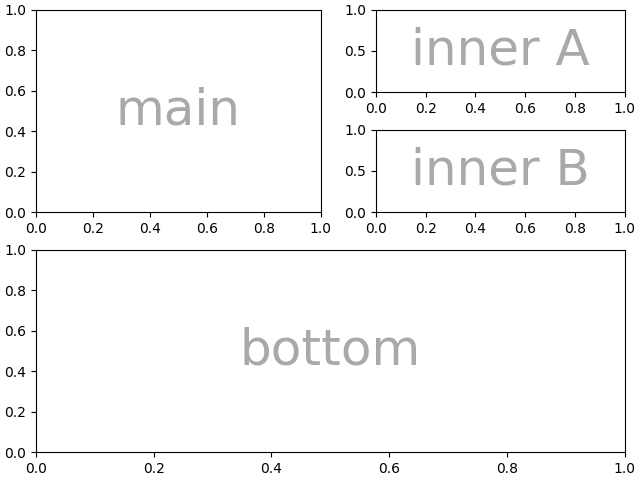We can also pass in a 2D NumPy array to do things like

mosaic = np.zeros((4, 4), dtype=int)
for j in range(4):
mosaic[j, j] = j + 1
axd = plt.figure(constrained_layout=True).subplot_mosaic(
mosaic,
empty_sentinel=0,
)
identify_axes(axd)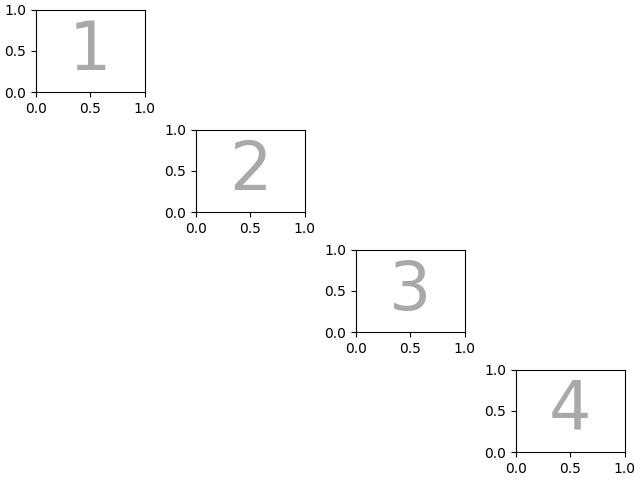Total running time of the script: ( 0 minutes 6.155 seconds)

Keywords: matplotlib code example, codex, python plot, pyplot Gallery generated by Sphinx-Gallery